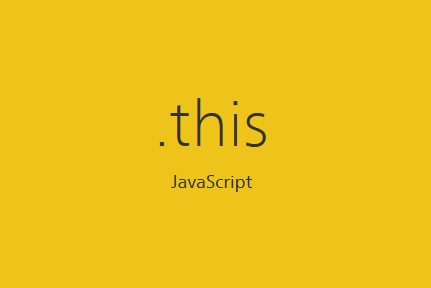# The 'this' Keyword in JavaScript

by Nicklas Envall

The keyword `this` is something Javascript developers kind of get, but not enough to be comfortable with it. So why not look at how `this` works so we can become more comfortable?`this` is a runtime binding. What `this` references depend on how a function is called, meaning the binding is created when the function is called.

Analysing the call-site helps us understand what `this` will reference. The call-site is the place in the code where a function is called (not declared). For example:

``````function greet() {
console.log("hello");
}

greet(); // This is a call-site for greet``````

## The four rules of This

Okay great, we now know that we should look at the call-site, but you can call a function in many different ways, right?

Luckily we have 4 rules for how a call-site would determine where `this` points to. In some cases multiple rules might apply, but we only use one, which is decided by priority:

`new > explicit > implicit > default`

### 1. Default Binding

``````function greet() {
console.log(this.x);
}

var x = "hello";
greet(); // hello``````

With default binding, `this` points to the global object. It's called default because if we haven't applied any of the other rules, default binding will occur. If we would have had `strict mode`, the global context would've been undefined.

### 2. Implicit Binding

``````function showAge() {
console.log(this.age);
}

var person = {
age: 45,
showAge: showAge
}

var age = 20;
person.showAge(); // 45``````

Here we can see that `this` uses the `person` object's context. If we analyse the call-site, we see that the object is used to reference the function when calling it. When we call the function in this way, the function's `this` becomes the object.

The following similar example might be confusing:

``````function showAge() {
console.log(this.age);
}

var person = {
age: 45,
showAge: showAge
}

var age = 30;
var showAge = person.showAge;

showAge(); // 30``````

You would assume that it would've been 45, but it's 30. The reason for this is that `person.showAge` is just a reference to the function `showAge`. Remember that we are interested in how we call the function.

### 3. Explicit Binding

With explicit binding, we can ensure that `this` points to what we want by using `call`, `apply`, or `bind`.

``````function foo() {
console.log(this.x);
}

var obj = {
x: 100
}

foo.call(obj); // 100``````

In the example above we use explicit binding to force `obj` to be the `this` in the `foo` function.

We can also hard bind, ES5 gives us Function.prototype.bind, which allows us to more easily hard bind.

``````function foo() {
console.log(this.x);
}

var obj = {
x: 100
}

var bar = foo.bind(obj);
bar(); // 100``````

If you think about it, using `bind` can also create some confusion. The example below looks similar to a default binding and someone might scratch their head for a long time wondering why it's logging 100 and not 13.

``````function foo() {
console.log(this.x)
}

var obj  = {
x: 100
}

var logX = foo.bind(obj);

// let's pretend we go 20 lines down

var x = 13;
logX(); // 100``````

### 4. new Binding

In Javascript, there are no specific constructor functions, but there are constructor calls. Calling a function with the keyword `new` in front of it makes it a constructor call. Commonly, we capitalize the name of a function to mark it as a constructor.

``````function Person(age) {
this.age = age;
}

var steve = new Person(50);
var jessica = new Person(27);

console.log(steve.age); // 50
console.log(jessica.age); // 27``````

Here we have constructed a new object and ensured that the new object is `this`.

## Binding this to Arrow Function

With ES6, we have arrow functions, which many of us like to use. But have you ever been confused why `this` does not work as you expect it would've when using arrow functions? Like getting `undefined` when using `this`?

Well, arrow functions do not follow the four rules they bind `this` based on scope in javascript. ES6 arrow functions use lexical scoping for determining `this`.

### Function vs Arrow Function

Lastly, I'll give you some code examples that show how a function and arrow function would work in some different scenarios.

#### Example 1

Let's start with an example of `this` with a normal function in an object:

``````var person = {
age: 40,
printAge: function() {
console.log(this.age)
}
}

person.printAge(); // 40``````

Now the exact same code, but with an arrow-function:

``````var person = {
age: 40,
printAge: () => {
console.log(this.age)
}
}

person.printAge(); // undefined``````

#### Example 2

Before you say "I'll never use arrow functions when creating methods then, I guess?", do consider the following code examples:

``````
var dollarStore = {
price: "1\$",
items: ["Orange", "Soda", "Apple"],

getItems: function() {
return this.items.map(function (item) {
return item + " costs " + this.price;
});
}
}

console.log(dollarStore.getItems()); // ["Orange costs undefined", "Soda costs undefined", "Apple costs undefined"]``````
``````var dollarStore = {
price: "1\$",
items: ["Orange", "Soda", "Apple"],

getItems: function() {
return this.items.map(item => item + " costs " + this.price);
}
}

console.log(dollarStore.getItems()); // ["Orange costs 1\$", "Soda costs 1\$", "Apple costs 1\$"]``````

If you want to avoid using an arrow-function in this scenario you could save `this` in a variable like:

``````var dollarStore = {
price: "1\$",
items: ["Orange", "Soda", "Apple"],

getItems: function() {
var that = this;
return this.items.map(function (item) {
return item + " costs " + that.price;
});
}
}

console.log(dollarStore.getItems()); // ["Orange costs 1\$", "Soda costs 1\$", "Apple costs 1\$"]
``````